Students can download 11th Business Maths Chapter 5 Differential Calculus Ex 5.3 Questions and Answers, Notes, Samcheer Kalvi 11th Business Maths Guide Pdf helps you to revise the complete Tamilnadu State Board New Syllabus, helps students complete homework assignments and to score high marks in board exams.

## Tamilnadu Samacheer Kalvi 11th Business Maths Solutions Chapter 5 Differential Calculus Ex 5.3

### Samacheer Kalvi 11th Business Maths Differential Calculus Ex 5.3 Text Book Back Questions and Answers

Question 1.
Examine the following functions for continuity at indicated points.Solution:
(a) f(x) = $$\frac{x^{2}-4}{x-2}$$, also given that f(2) = 0[∵ x = 2 – h, where h → 0, x → 2]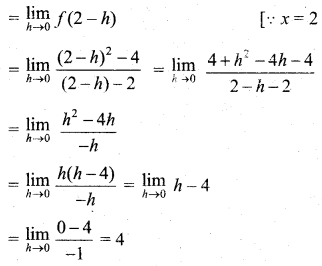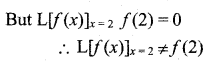∴ The given function is not continuous at x = 2.(b) Given that f(x) = $$\frac{x^{2}-9}{x-3}$$ and f(3) = 6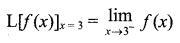[∵ x = 3 – h, where h → 0, x → 3]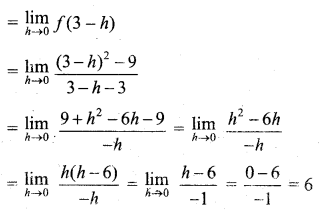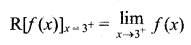[∵ x = 3 + h, where x → 3, h → 0]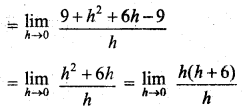= 0 + 6
= 6
Also given that f(3) = 6∴ The given function f(x) is continuous at x = 3.Question 2.
Show that f(x) = |x| is continuous at x = 0.
Solution: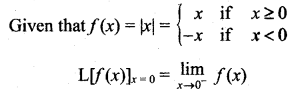[∵ x = 0 – h]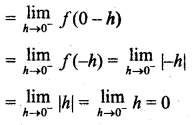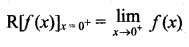[∵ |x| = x if x > 0]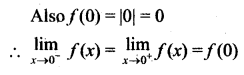∴ f(x) is continuous at x = 0.